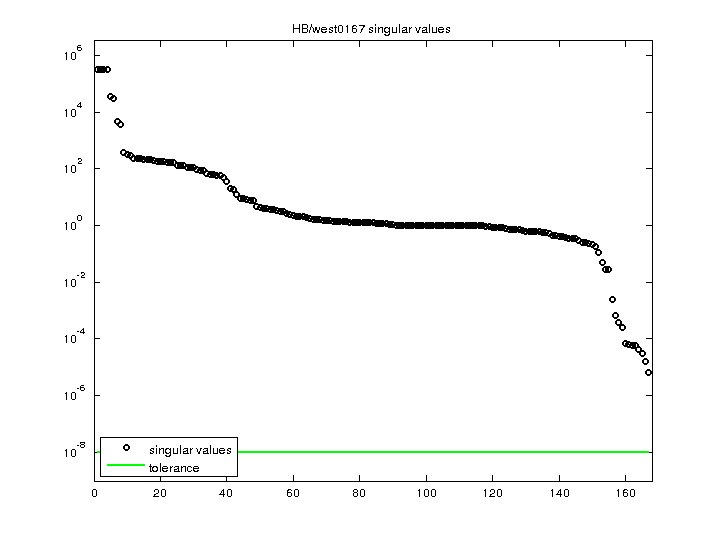Matrix: HB/west0167

Description: U RIGOROUS MODEL OF A CHEMICAL STAGE (CHEM.ENG. FROM A. WESTERBERG)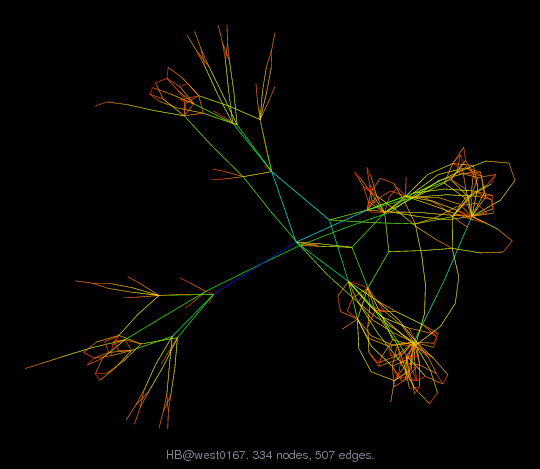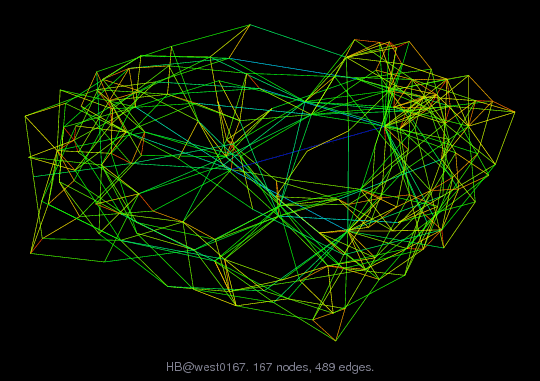(bipartite graph drawing) (graph drawing of A+A')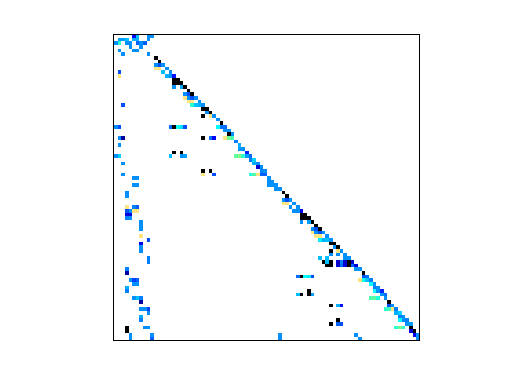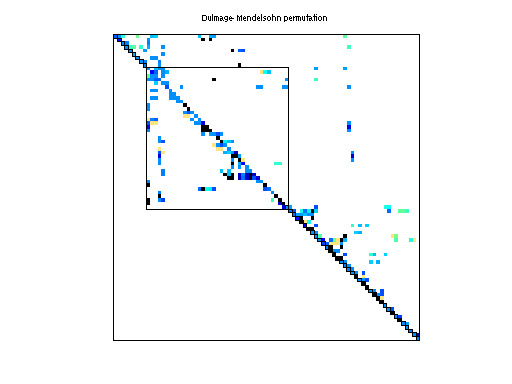• Matrix group: HB
• download as a MATLAB mat-file, file size: 4 KB. Use UFget(265) or UFget('HB/west0167') in MATLAB.

 Matrix properties number of rows 167 number of columns 167 nonzeros 506 structural full rank? yes structural rank 167 # of blocks from dmperm 91 # strongly connected comp. 1 explicit zero entries 1 nonzero pattern symmetry 2% numeric value symmetry 0% type real structure unsymmetric Cholesky candidate? no positive definite? no

 author A. Westerberg editor I. Duff, R. Grimes, J. Lewis date 1983 kind chemical process simulation problem 2D/3D problem? no

 Ordering statistics: result nnz(chol(P*(A+A'+s*I)*P')) with AMD 2,039 Cholesky flop count 3.6e+04 nnz(L+U), no partial pivoting, with AMD 3,911 nnz(V) for QR, upper bound nnz(L) for LU, with COLAMD 470 nnz(R) for QR, upper bound nnz(U) for LU, with COLAMD 1,049

Note that all matrix statistics (except nonzero pattern symmetry) exclude the 1 explicit zero entries.

 SVD-based statistics: norm(A) 320116 min(svd(A)) 6.68968e-06 cond(A) 4.78522e+10 rank(A) 167 sprank(A)-rank(A) 0 null space dimension 0 full numerical rank? yes

 singular values (MAT file): click here SVD method used: s = svd (full (A)) ; status: ok